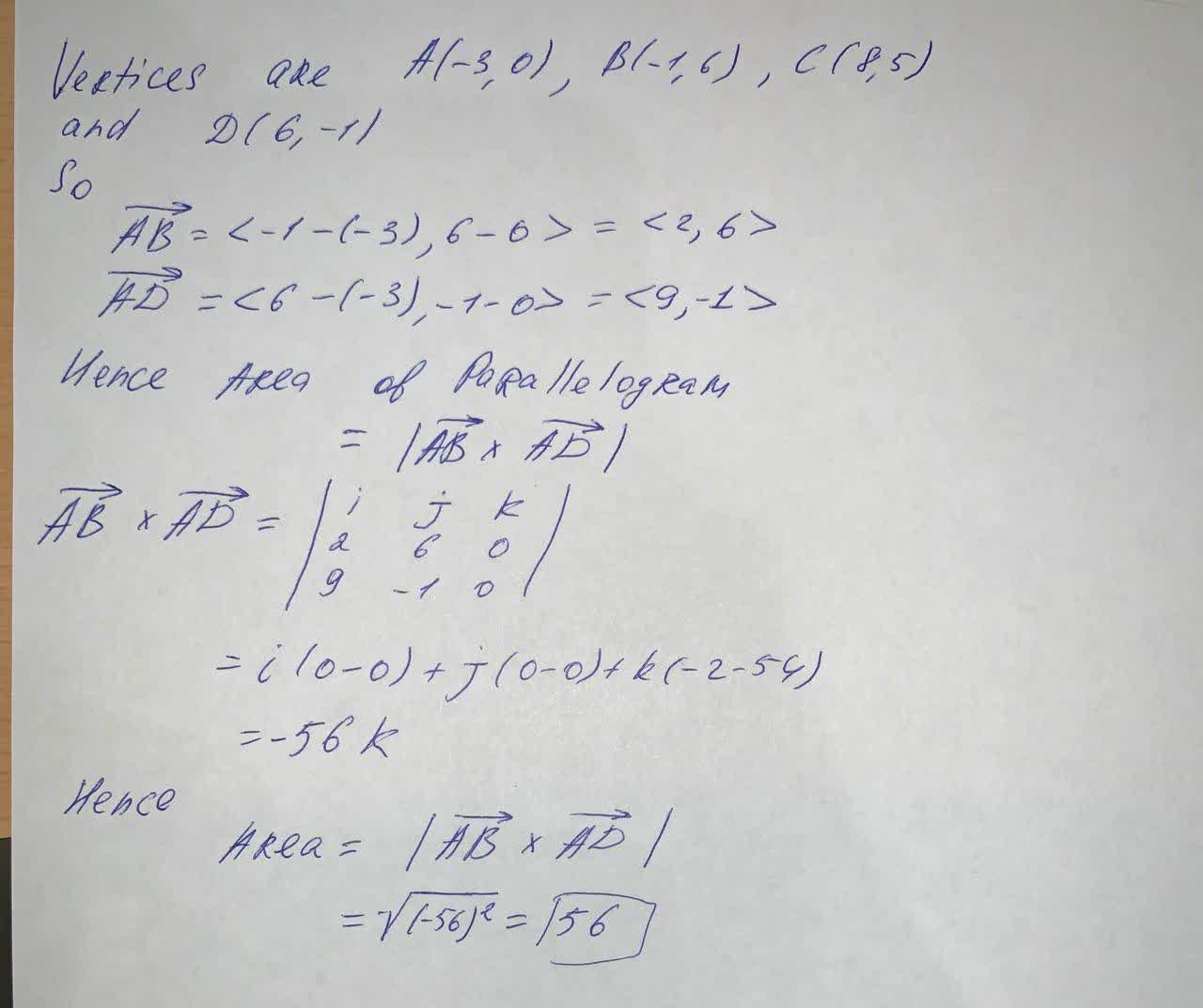Find the area of the parallelogram with vertices A(-3,0) , B(-1,6) , C(8,5) and D(6,-1)Reeves 2021-05-13 Answered
Find the area of the parallelogram with vertices A(-3,0) , B(-1,6) , C(8,5) and D(6,-1)

• Questions are typically answered in as fast as 30 minutes

Solve your problem for the price of one coffee

• Math expert for every subject
• Pay only if we can solve itCristiano Sears

See the answer below:​​​Not exactly what you’re looking for?content_user

Vertices are $$A(-3,0),\ B(-1,6),\ C(8,5)$$ and $$D(6,-1)$$

So

$$\vec{AB}=<-1-(-3),6-0>=<2,6>$$

$$\vec{AD}=<6-(-3),-1-0>=<9,-1>$$

Hence area of parallelogram

$$=|\vec{AB}\times\vec{AD}|$$

$$\vec{AB}\times\vec{AD}=\begin{bmatrix}i&j&k\\2&6&0\\9&-1&0\end{bmatrix}$$

$$=i(0-0)+j(0-0)+k(-2-54)$$

$$=-56k$$

Hence

Area  $$=|\vec{AB}\times\vec{AD}|$$

$$=\sqrt{(-56)^2}=56$$